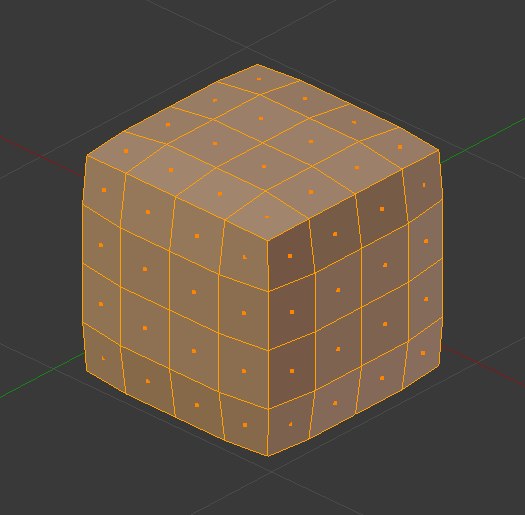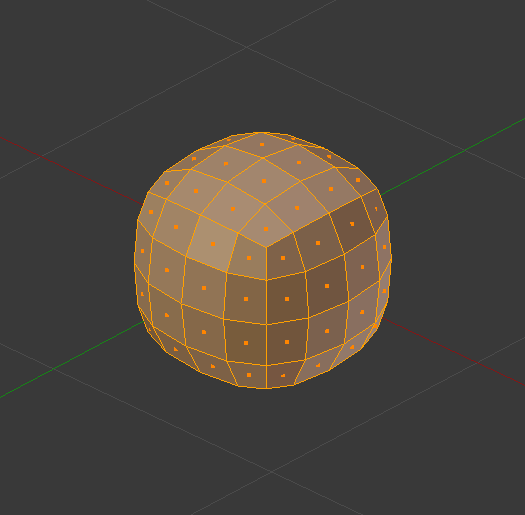# Smooth Modifier¶

The Smooth modifier smooths a mesh by flattening the angles between adjacent faces in it, just like the Smooth tool in Edit Mode. It smooths without subdividing the mesh, the number of vertices remains the same.

This modifier is not limited to smoothing, though. Its control factor can be configured outside the (0.0 to 1.0) range (including negative values), which can result in interesting deformations.

## Options¶The Smooth modifier.

Axis

Enable/disable the modifier in the X, Y and/or Z axes directions.

Factor

Controls the smoothing amount. Higher values will increase the effect.

Values outside expected range (above 1.0 or below 0.0) will distort the mesh.

Repeat

The number of smoothing iterations, equivalent to executing the Smooth tool multiple times.

Vertex Group

If set, restrict the effect to the only vertices in that vertex group. This allows for selective, real-time smoothing, by painting vertex weights.

Invert <->

Inverts the influence of the selected vertex group, meaning that the group now represents vertices that will not be deformed by the modifier.

The setting reverses the weight values of the group.

## Algorithm¶

The calculation done by the Smooth modifier is a simple and logical one, and can be thought of as the geometric equivalent of blurring images.

Each new vertex position is simply moved towards the average position of all its neighbor vertices (topologically speaking, i.e. the vertices directly connected to it by an edge).

## Example¶Mesh after one smoothing iteration.¶Mesh after ten smoothing iterations.¶